# RS Aggarwal Class 9 Solutions Chapter 1 Real Numbers Ex 1B

## RS Aggarwal Class 9 Solutions Chapter 1 Real Numbers Ex 1B

These Solutions are part of RS Aggarwal Solutions Class 9. Here we have given RS Aggarwal Solutions Class 9 Chapter 1 Real Numbers Ex 1B.

Other Exercises

Question 1.
Solution:
We know that a fraction $$\frac { p }{ q }$$ is terminating if prime factors of q are 2 and 5 only.
Hence.
(i) $$\frac { 13 }{ 80 }$$ and $$\frac { 16 }{ 125 }$$ are the terminating decimals.

Question 2.
Solution: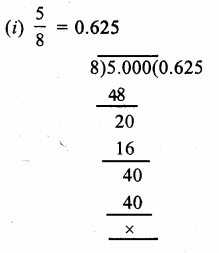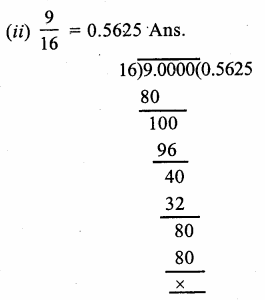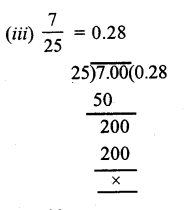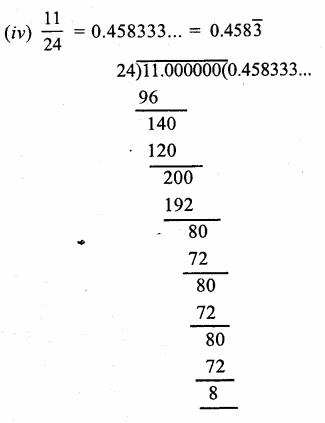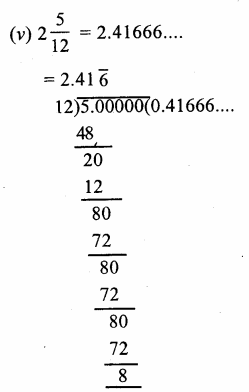Question 3.
Solution:
(i) Let,x = 0.$$\overline { 3 }$$ = 0.3333…(i)
Then, 10x = 3.3333….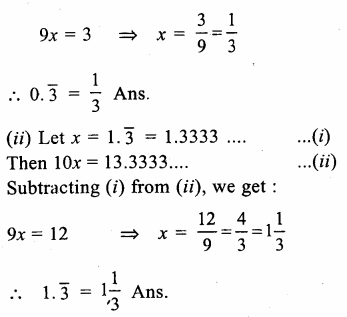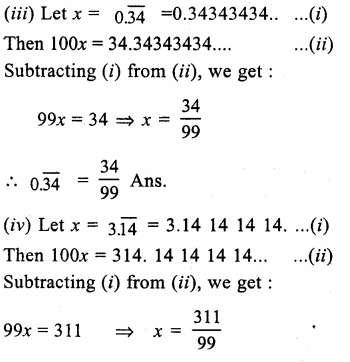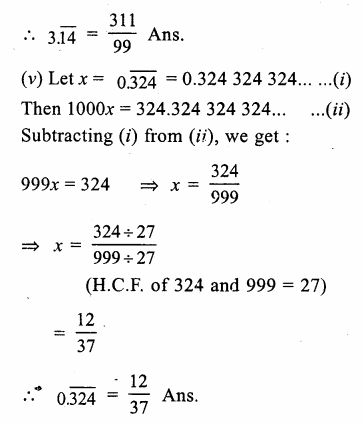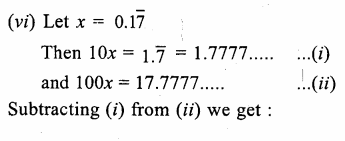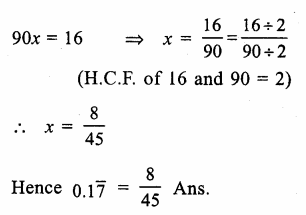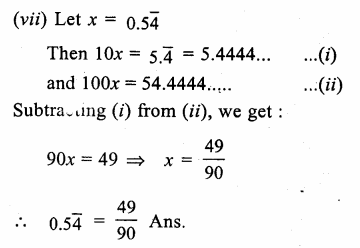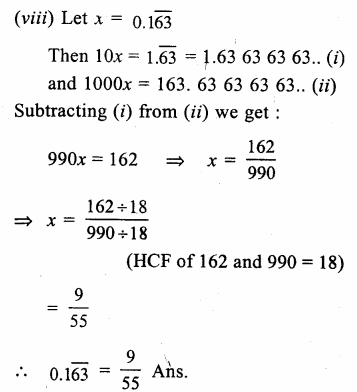Question 4.
Solution:
(i) True, because set of natural numbers is a subset of whole number.
(ii) False, because the number 0 does not belong to the set of natural numbers.
(iii) True, because a set of integers is a subset of a rational numbers.
(iv) False, because the set of rational numbers is not a subset of whole numbers.
(v) True, because rational number can be expressed as terminating or repeating decimals.
(vi) True, because every rational number can be express as repeating decimals.
(vii) True, because 0 = $$\frac { 0 }{ 1 }$$, which is a rational number Ans.

Hope given RS Aggarwal Solutions Class 9 Chapter 1 Real Numbers Ex 1B are helpful to complete your math homework.

If you have any doubts, please comment below. Learn Insta try to provide online math tutoring for you.